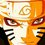# Daily Note #1 - Fermat's Little Theorem

So, starting with my first daily note, the topic is Fermat's Little Theorem.

Topic: Number Theory

Fermat's Little Theorem states that

"For all natural numbers 'a' , $$a^{p} \equiv a \mod p$$ , where 'p' is a prime number. "

Let us prove this out.

Consider the binomial expansion for the prime 'p',

$(a+b)^{p} = a^{p} + \binom{p}{1} a^{p-1} b +\binom{p}{2} a^{p-2} b^{2} + ....... + b^{p}$.

But since, $p| \binom{p}{k} \forall k=1,2,3,.....p-1$. So, $\binom{p}{1} a^{p-1} b +\binom{p}{2} a^{p-2} b^{2} + ....... + \binom{p}{p-1} a b^{p-1} = Multiple(p)$. This implies that, $(a+b)^{p} \equiv a^{p} + b^{p} \mod p$.

Generalizing this we get, $(a_{1}+a_{2} +.....a_{n})^{p} \equiv a_{1}^{p} + a_{2}^{p} +........ +a_{n}^{p} \mod p$. By taking $a_{1}=a_{2}=.....=a_{n}=1$, we get $n^{p} \equiv n \mod p$. That's it, we got the result.

Phewwwwwww!! We have proved it.

Fermat's Theorem is very useful in some problems based on Modular Arithmetic.

Now, if $(a,p) =1$,i.e. if $a$ and $p$ are coprime to each other, then $a^{p-1} \equiv 1 \mod p$. This is known as Fermat's Little Theorem and it is a special case of Euler's Totient Theorem.

Now let us solve some problems.

Problem 1(introductory): Find the remainder when $37^{1123}$ is divided by $17$.

Solution: Observe that $37 \equiv 3 \mod 17$ and from Fermat's Theorem $37^{17} \equiv 37 \mod 17$. But $1123 = 66 \times 17 + 1$. So, $37^{1123} \equiv (37^{17})^{66} \times 37 \equiv 37^{66} \times 37 \equiv 37^{67} \equiv (37^{17})^{3} \times 37^{16}$ $\equiv 37^{3} \times 37^{16} \equiv 37^{17} \times 37^{2} \equiv 37 \times 37^{2} \equiv 37^{3} \equiv 3^{3} \equiv 27 \equiv 10 \mod 17$.

So, $37^{1123} \equiv 10 \mod 17$. This is can be even very easily using Fermat's littile theorem(Try Yourselves).

Problem 2: Find the remainder when $2^{20} + 3^{30} + 4^{40} + 5^{50} + 6^{60}$ is divided by $7$.

Solution: Observe that $(2,7)=(3,7)=(4,7)=(5,7)=(6,7)=1.$ So, $2^{20} \equiv 2^{2} \mod 7$, $3^{30} \equiv 1 \mod 7$, $4^{40} \equiv 4^{4} \equiv 4 \mod 7$, $5^{50} \equiv 5^{2} \equiv 4 \mod 7$ and $6^{60} \equiv 1 \mod 7$. So, $2^{20} + 3^{30} + 4^{40} + 5^{50} + 6^{60} \equiv 4+1+4+4+1 \equiv 0 \mod 7$.

So, $2^{20} + 3^{30} + 4^{40} + 5^{50} + 6^{60} \equiv 0 \mod 7$.

So, I think that I have given a clear picture on Fermat's Theorem. So, stay tuned for upcoming DAILY NOTES.Note by Surya Prakash
5 years, 9 months ago

This discussion board is a place to discuss our Daily Challenges and the math and science related to those challenges. Explanations are more than just a solution — they should explain the steps and thinking strategies that you used to obtain the solution. Comments should further the discussion of math and science.

When posting on Brilliant:

• Use the emojis to react to an explanation, whether you're congratulating a job well done , or just really confused .
• Ask specific questions about the challenge or the steps in somebody's explanation. Well-posed questions can add a lot to the discussion, but posting "I don't understand!" doesn't help anyone.
• Try to contribute something new to the discussion, whether it is an extension, generalization or other idea related to the challenge.

MarkdownAppears as
*italics* or _italics_ italics
**bold** or __bold__ bold
- bulleted- list
• bulleted
• list
1. numbered2. list
1. numbered
2. list
Note: you must add a full line of space before and after lists for them to show up correctly
paragraph 1paragraph 2

paragraph 1

paragraph 2

[example link](https://brilliant.org)example link
> This is a quote
This is a quote
    # I indented these lines
# 4 spaces, and now they show
# up as a code block.

print "hello world"
# I indented these lines
# 4 spaces, and now they show
# up as a code block.

print "hello world"
MathAppears as
Remember to wrap math in $$ ... $$ or $ ... $ to ensure proper formatting.
2 \times 3 $2 \times 3$
2^{34} $2^{34}$
a_{i-1} $a_{i-1}$
\frac{2}{3} $\frac{2}{3}$
\sqrt{2} $\sqrt{2}$
\sum_{i=1}^3 $\sum_{i=1}^3$
\sin \theta $\sin \theta$
\boxed{123} $\boxed{123}$

Sort by:

Is there anything that you can add to the Fermat's Little Theorem Wiki page?

Staff - 5 years, 9 months ago

Another way to prove Fermat's Theorem by using reduced residue system !!

Given that : { ${ r_{1},r_{2},...,r_{p-1} }$ } is the reduced residue system of p

Because of $(a,p) = 1$ so $(a.r_{i},p) = 1; (i = 1,2,...,p-1 )$

So that : $a.r_{1}.a.r_{2}...a.r_{p-1} \equiv r_{1}.r_{2}...r_{p-1} \pmod{p}$

$\Rightarrow a^{p-1}.r_{1}.r_{2}...r_{p-1} \equiv r_{1}.r_{2}...r_{p-1} \pmod{p}$

Thus,

$a^{p-1} \equiv 1 \pmod{p}$

The theorem is proved !!

- 5 years, 9 months ago

That is how I've been doing it yet.

- 3 years, 3 months ago

Nice work, bro. Upvoted $\ddot \smile$.

- 5 years, 9 months ago

Tks, i have checked your profile, it very impress, proud to be a vietnamese :D

- 5 years, 9 months ago

Yep, proud to be Vietnamese :)

- 5 years, 9 months ago